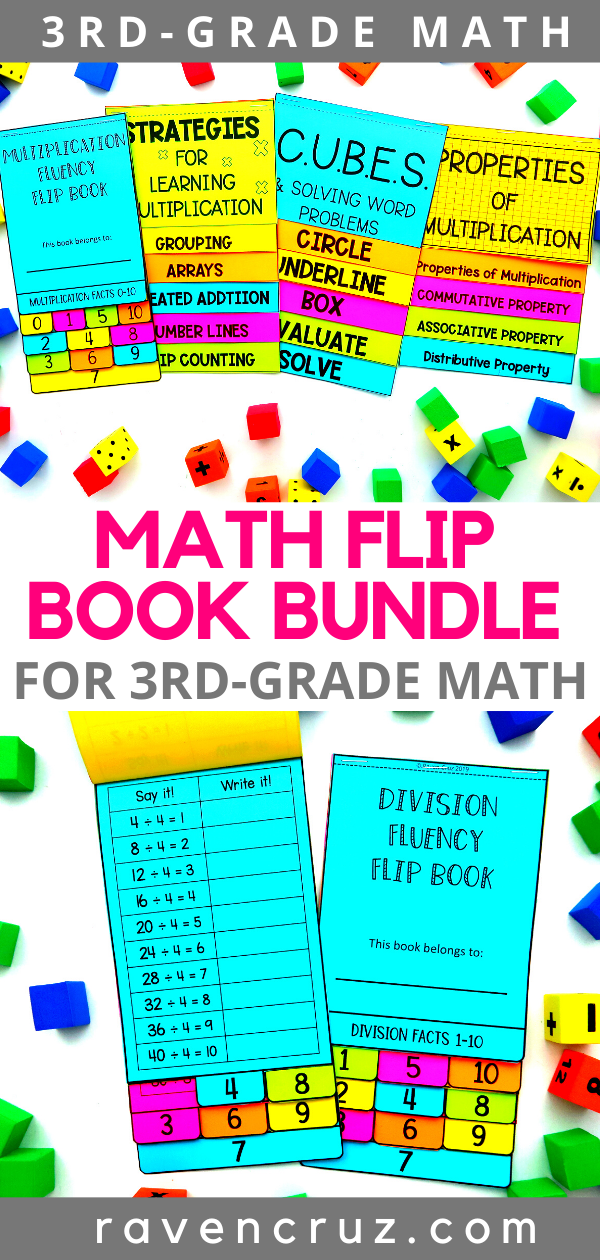Math Flip Books for 3rd-Grade - Raven Cruz

# Math Flip Books for 3rd-Grade

Flip books make me HAPPY! Math flip books for 3rd-grade make me SUPER HAPPY

I don't know about you, but I get excited when I know it is flip book construction day. First of all, students are always excited when they see flip books. Second of all, students' happiness is contagious and helps get me excited. Last, flip books are great reference tools for interactive notebooks and even math toolboxes.

So what doe the flip books cover?

## Multiplication Strategies

Multiplication strategies covered with the flip book include: equal groups, arrays, repeated addition, number lines, skip counting.

Each strategy has 3 examples and a definition. For example, equal groups includes a model of 4 equal groups of 4, explains that "4 groups of 4 equals 16", and a number sentence that explains 4 x 4 = 16 (see the photo above).

This flip book is the #1 BEST SELLER in my Teachers Pay Teachers store!

## Multiplication Fluency

The multiplication fluency flip books have been constructed to introduce multiplication facts in the order in which I prefer to teach them. Based on the study of teaching the easiest facts first; 0, 1, 5, and 10. Next, 2, 4, and 8. Next, 3, 6, and 9. Last, the facts for 7 (see the photo above).

Students are asked to say the multiplication sentence then, write the multiplication sentence. In this step you could also encourage you students to use the commutative property to write the multiplication sentence in another way.

The multiplication fluency flip book covers facts 0-10 and recently added by request, facts 0-12.

This flip book is the SECOND BEST SELLER in my Teachers Pay Teachers store (which is amazing because it is one of my newer resources).

## C.U.B.E.S Math Strategy

C.U.B.E.S. math strategy is commonly used to teach students how to deconstruct word problems to make them easier to solve.

Circle all the important numbers, underline the question, box all the important math words, evaluate the important words that you may have left out and eliminate what you don't need, and solve the word problem.

This flip book is a compact way for students to review the steps at home or at school. Plus, I always think providing parents with manipulatives to help their kiddos reinforce what they are being taught is a FABULOUS THING! AND this flip book is not 3rd-grade specific so it can be used for many years.

## Properties of Multiplication

My personal favorite flip book, and the most difficult for me to create is the properties of multiplication flip book. This flip book covers the commutative property of multiplication, associative property of multiplication, and the distributive property of multiplication.

Each flap includes a definition, example, and a place for students to finish the problem (see photo above).

This flip book is the THIRD BEST SELLER (out of over 200 resources) in my Teachers Pay Teachers store.

## Division Fluency

Like the multiplication fluency flip books, the division fluency flip books have been constructed to introduce division facts in the order in which I prefer to teach them. Based on the study of teaching the easiest facts first; 1, 5, and 10. Next, 2, 4, and 8. Next, 3, 6, and 9. Last, the facts for (see the photo above).

Students are asked to say the division sentence then, write the division sentence.

## Coming Soon:  Division Strategies